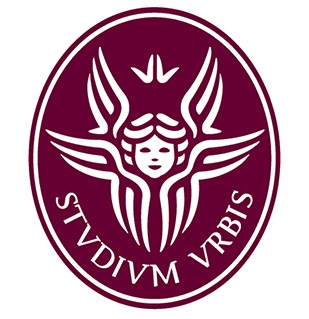Gabriella Puppo Dipartimento di Matematica
La Sapienza Universita' di Roma
Piazza Aldo Moro 5
00185 Roma
ItalyNumerical integration of Partial Differential Equations, especially of hyperbolic type. Models of mathematical physics.

• Finite difference and finite volume methods for conservation and balance laws, with particular emphasis on high order methods, stiff problems and on the preservation of equilibrium solutions.
• Kinetic models: Models for mixture of gases, Polyatomic gases, Traffic models.
• Methods for kinetic problems: noise reduction for Monte Carlo methods, domain decomposition and multiscale methods, numerical integration of simplified models, such as the BGK equation.
• Adaptive and multiscale methods: a-posteriori error indicator based on entropy production for hyperbolic problems, domain decomposition methods for kinetic models. Preservation of asymptotic and equilibrium states in numerical schemes.
• Relaxation methods for systems of equations.
• Spectral methods and finite element methods: stabilization of convection diffusion equations.
• Vortex methods for incompressible equations.
She authors or co-authors more than fifty scientific publications, appeared on the best international journals of the field: J. Comp. Phys, SIAM J. Scientific Computing, J. Scientific Computing, SIAM J. of Numerical Analysis, Communications in Computational Physics, Math. of Comp., Computers and Fluids, Kinetics and Related Models, Communications in Math. Sciences.